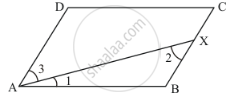Advertisement Remove all ads

# In a Parallelogram Abcd, the Bisector of ∠A Also Bisects Bc at X. Find Ab : Ad. - Mathematics

Answer in Brief

In a parallelogram ABCD, the bisector of ∠A also bisects BC at X. Find AB : AD.

Advertisement Remove all ads

#### Solution

Parallelogram ABCD is given as follows:We have AX bisects  ∠A bisecting BC at X.

That is,  BX = CX

We need to find AB :AD

Since, AX is the bisector ∠A

That is,

∠1 =1/2 ∠A  …… (i)

Also, ABCD is a parallelogram

Therefore, AD || BC and AB intersects them

∠A +∠B = 180°

∠B = 180° - ∠A …… (ii)

In ΔABX,by angle sum property of a triangle:

∠1 +∠2 + ∠B = 180°

From (i) and (ii), we get:

1/2 ∠A + ∠2 + 180° - ∠A = 180°

∠2- 1/2 ∠A = 0

∠2 = 1/2 ∠A …… (iii)

From (i) and (iii),we get:

∠1 = ∠2

Sides opposite to equal angles are equal. Therefore,

BX = AB

2BX = 2AB

As X is the mid point of BC. Therefore,

BC = 2AB

Also, ABCD is a parallelogram, then,  BC = AD

AD = 2AB

Thus,

AB :AD = AB :2AB

AB :AD = 1:2

Hence the ratio of AB : AD is 1:2.

Is there an error in this question or solution?
Advertisement Remove all ads

#### APPEARS IN

RD Sharma Mathematics for Class 9
Chapter 13 Quadrilaterals
Q 10 | Page 69
Advertisement Remove all ads

#### Video TutorialsVIEW ALL 

Advertisement Remove all ads
Share
Notifications

View all notifications

Forgot password?